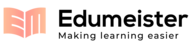## 2010_Term_test_1_sol.pdf-University of Toronto Division of Engineeringshowing page 1-2 out of 7

##### Page 1
1
University of Toronto
Division of Engineering Science
STA286S: Probability and Statistics
Term Test - Solution
Monday, March 1, 2010, 9:10-11am
Examiners: V. Makis and H. Moshonov
Instructions:
Time allowed:
1 hour and 50 minutes.
Aids:
a non-programmable calculator and a two sided A4 size aid sheet.
This test may be long, so be sure to proportion your time carefully among the questions and limit
your time spent on any single question.
If you do not understand a question, or are having some other difficulty, do not hesitate to ask your
instructor or T.A for clarification.
Marks are shown in brackets at the beginning of each question. Total points: 100.
GOOD LUCK
Question
1
2
3
4
5
6
7
Total
Max
10
13
10
17
10
20
20
100
Score

##### Page 2
2
Question 1
Suppose two dice are tossed and the numbers on the upper faces are observed. Let
A
be the event that
the number on the second die is even and let
B
be the event that the sum of the two numbers is even.
a)
(4 marks) Describe the sample space
S
.
There are 36 elements in the sample space as follow:
(
)
(
)
(
)
(
)
(
)
(
)
(
)
(
)
(
)
=
6
,
6
2
,
6
1
,
6
6
,
2
,
2
,
2
1
,
2
6
,
1
2
,
1
1
,
1
L
M
M
M
M
L
L
S
b)
(6 marks) List all the outcomes in
'
A
and
'
B
A
.
The event
'
A
is the event that the number on the second die is odd. The outcomes in
'
A
are
(
)(
)(
)(
)(
)(
)
(
)
(
)
(
)
(
)
(
)
(
)
(
)
(
)(
)(
)
(
)
(
)
{
}
5
,
6
,
3
,
6
,
1
,
6
,
5
,
5
,
3
,
5
,
1
,
5
,
5
,
4
,
3
,
4
,
1
,
4
,
5
,
3
,
3
,
3
,
1
,
3
,
5
,
2
,
3
,
2
,
1
,
2
,
5
,
1
,
3
,
1
,
1
,
1
'
=
A
The event
'
B
A
is the event that the outcome on the second die is event and the sum of the
two numbers is odd. It consist the following outcomes.
(
)(
)(
)(
)(
)
(
)
(
)
(
)
(
)
{
}
6
,
5
,
4
,
5
,
2
,
5
,
6
,
3
,
4
,
3
,
2
,
3
,
6
,
1
,
4
,
1
,
2
,
1
'
=
B
A
Question 2
Assume that eligible voters in Ontario have the following (federal) party preferences:
45% ‘Liberal’, 15% ‘Conservative’, 20% ‘Alliance’, 5% ‘NDP’, 15% ‘none of the above’
a)
(2 mark) Find the probability that an eligible voter will not be a Conservative supporter.
P(not conservative) = 1- P(conservative) = 1- 0.15 = 0.85.
b)
(3 marks) If we randomly choose two people from the eligible Ontario voters, find the probability
that our sample will include at least one Conservative supporter.
Let
A
be the event that the first person chosen is Conservative and
B
the event that the second
person chosen is Conservative, we have that
A
and
B
are independent so
(
)
(
)
(
)
(
)
(
)
(
)
(
)
2775
.
0
15
.
0
15
.
0
15
.
0
ve
conservati
one
least
at
2
=
+
=
+
=
=
B
P
A
P
B
P
A
P
B
A
P
P
c)
(3 marks) If we randomly choose three people from the eligible Ontario voters. Find the probability
that exactly one of these three will be a Liberal supporter.
Let
A
,
B
and
C
be the events that the first, second and third person chosen is Liberal
respectively. We have that
A
,
B
and
C
are independent, so
(
)
(
)
(
)
(
)
(
)(
)
4084
.
0
45
.
0
1
45
.
0
3
Liberal
one
exactly
2
=
=
+
+
=
C
B
A
P
C
B
A
P
C
B
A
P
P